# Graphs and Social Networks Why Social Graphs Are

• Slides: 13
Download presentationGraphs and Social Networks Why Social Graphs Are Different Communities Finding Triangles Jeffrey D. Ullman Stanford University/Infolab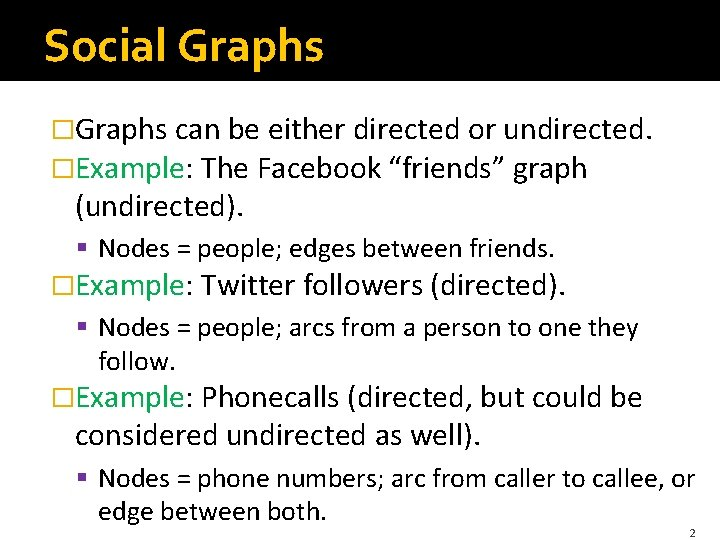Social Graphs �Graphs can be either directed or undirected. �Example: The Facebook “friends” graph (undirected). § Nodes = people; edges between friends. �Example: Twitter followers (directed). § Nodes = people; arcs from a person to one they follow. �Example: Phonecalls (directed, but could be considered undirected as well). § Nodes = phone numbers; arc from caller to callee, or edge between both. 2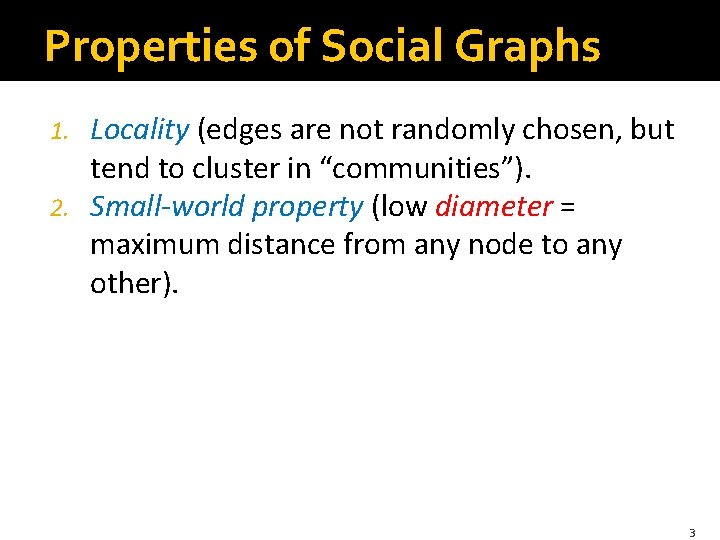Properties of Social Graphs Locality (edges are not randomly chosen, but tend to cluster in “communities”). 2. Small-world property (low diameter = maximum distance from any node to any other). 1. 3Locality �A graph exhibits locality if when there is an edge from x to y and an edge from y to z, then the probability of an edge from x to z is higher than one would expect given the number of nodes and edges in the graph. �Example: On Facebook, if y is friends with x and z, then there is a good chance x and z are friends. �Community = set of nodes with an unusually high density of edges. 4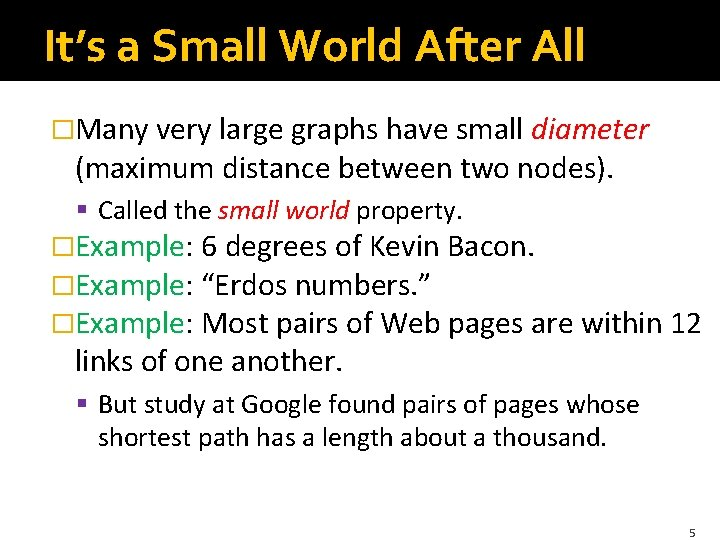It’s a Small World After All �Many very large graphs have small diameter (maximum distance between two nodes). § Called the small world property. �Example: 6 degrees of Kevin Bacon. �Example: “Erdos numbers. ” �Example: Most pairs of Web pages are within 12 links of one another. § But study at Google found pairs of pages whose shortest path has a length about a thousand. 5Finding Triangles Heavy Hitters Two Kinds of Triangles Optimal Algorithm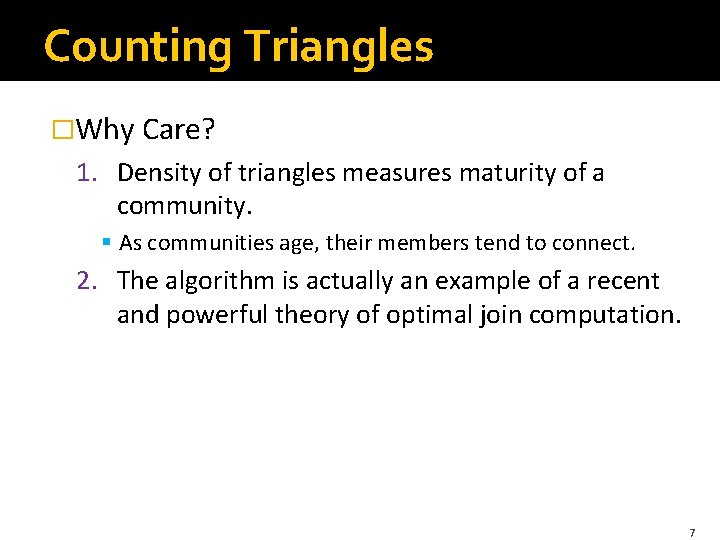Counting Triangles �Why Care? 1. Density of triangles measures maturity of a community. § As communities age, their members tend to connect. 2. The algorithm is actually an example of a recent and powerful theory of optimal join computation. 7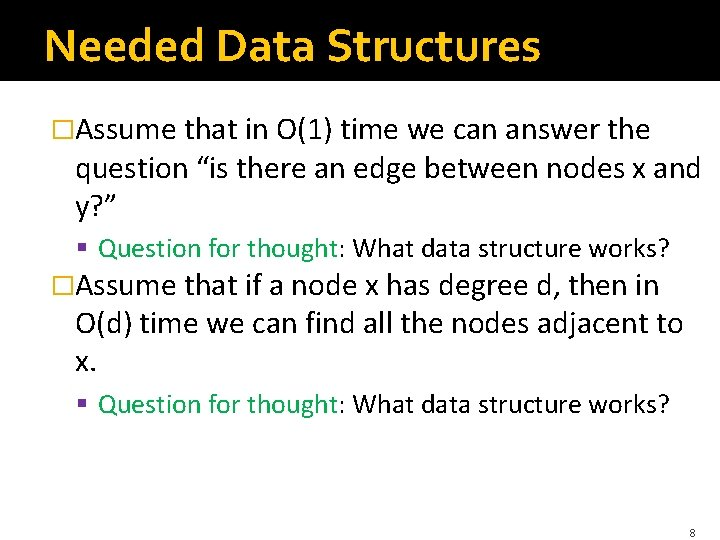Needed Data Structures �Assume that in O(1) time we can answer the question “is there an edge between nodes x and y? ” § Question for thought: What data structure works? �Assume that if a node x has degree d, then in O(d) time we can find all the nodes adjacent to x. § Question for thought: What data structure works? 8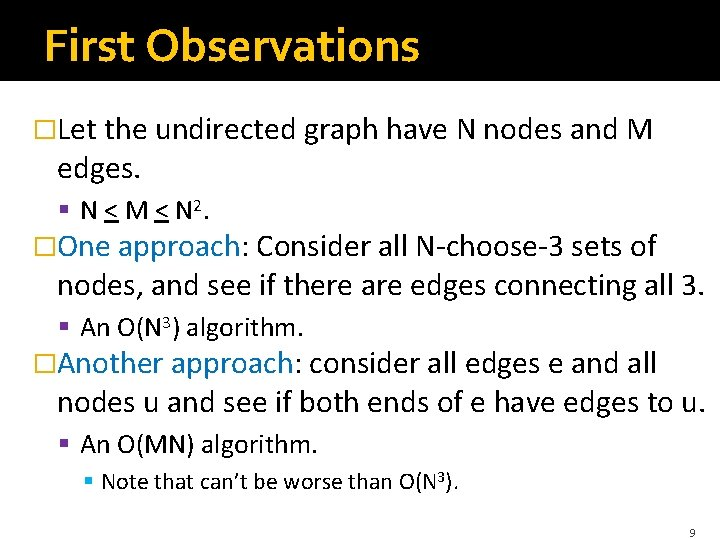First Observations �Let the undirected graph have N nodes and M edges. § N < M < N 2. �One approach: Consider all N-choose-3 sets of nodes, and see if there are edges connecting all 3. § An O(N 3) algorithm. �Another approach: consider all edges e and all nodes u and see if both ends of e have edges to u. § An O(MN) algorithm. § Note that can’t be worse than O(N 3). 9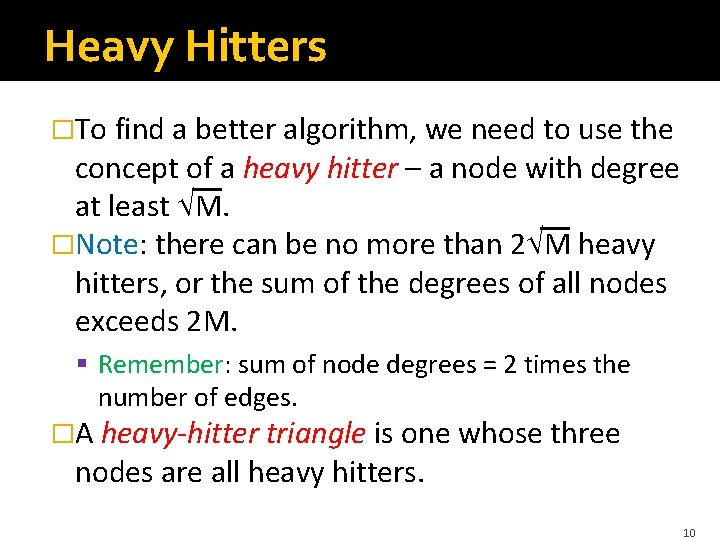Heavy Hitters �To find a better algorithm, we need to use the concept of a heavy hitter – a node with degree at least M. �Note: there can be no more than 2 M heavy hitters, or the sum of the degrees of all nodes exceeds 2 M. § Remember: sum of node degrees = 2 times the number of edges. �A heavy-hitter triangle is one whose three nodes are all heavy hitters. 10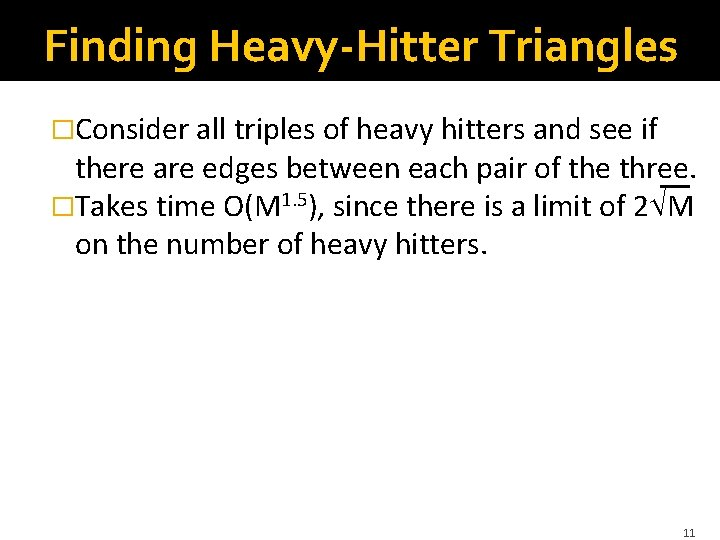Finding Heavy-Hitter Triangles �Consider all triples of heavy hitters and see if there are edges between each pair of the three. �Takes time O(M 1. 5), since there is a limit of 2 M on the number of heavy hitters. 11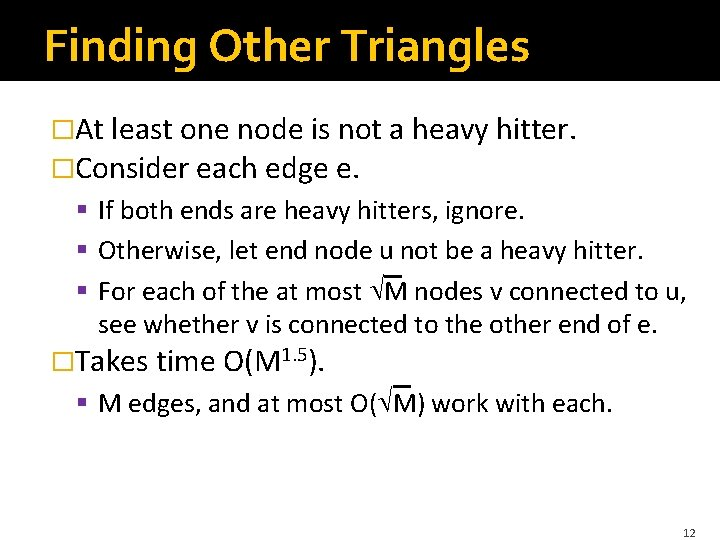Finding Other Triangles �At least one node is not a heavy hitter. �Consider each edge e. § If both ends are heavy hitters, ignore. § Otherwise, let end node u not be a heavy hitter. § For each of the at most M nodes v connected to u, see whether v is connected to the other end of e. �Takes time O(M 1. 5). § M edges, and at most O( M) work with each. 12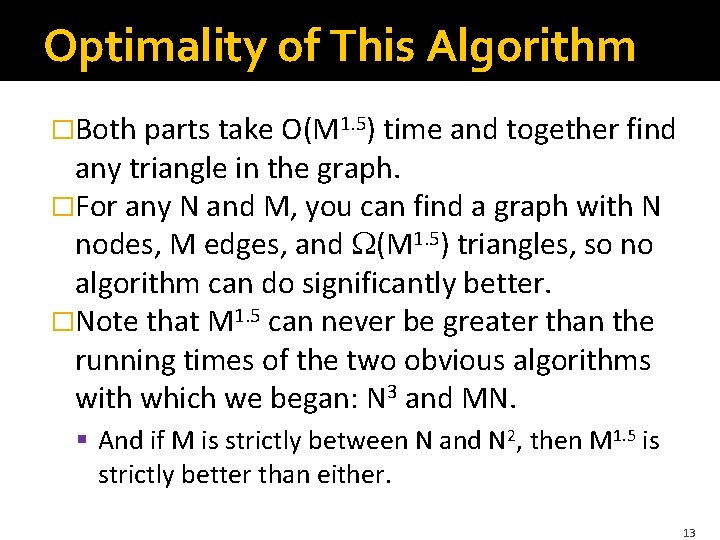Optimality of This Algorithm �Both parts take O(M 1. 5) time and together find any triangle in the graph. �For any N and M, you can find a graph with N nodes, M edges, and (M 1. 5) triangles, so no algorithm can do significantly better. �Note that M 1. 5 can never be greater than the running times of the two obvious algorithms with which we began: N 3 and MN. § And if M is strictly between N and N 2, then M 1. 5 is strictly better than either. 13